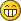I never used ROTSTEP, but about the question "Also does anyone know the best/easiest way to extract the angles from an existing rotation matrix?", attached you can find the Excel file I use to convert angles to matrix and vice versa (second sheet), plus some useful trigonometric formulas to estimate the angles to be applied to pieces (first sheet).

You can paste in B2 the rotation matrix copied from LDCad and see the results in the B1315 range (choose the axis sequence you prefer), or obtain the resulting rotation matrix in B28, from the angles applied in the x-z-y order from the B19:B21 cells.

Note that the 2D arctangent in Excel uses the arguments in reversed order in respect to other programming languages and math bibliography.

I don't know if this is the easiest/best way to obtain them, I have no PhD in mathematics :-P
Ignore the round formula.

EDIT:
Oops, just saw you already found a solution.. I have not yet studied your formulas, but I have not seen a check condition for the +/-90 degrees singularity. Have you checked the rotation matrix 0 -1 0 1 0 0 0 0 1?
« Next Oldest | Next Newest »